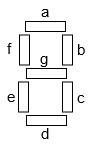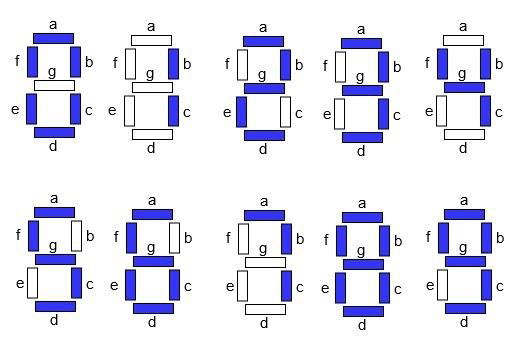# Count changes in Led Lights to display digits one by one in C++

C++Server Side ProgrammingProgramming

We are given a positive number let’s say num and the task is to calculate the count of changes in the Led lights displayed one by one. We will assume that initially all LEDs are off and they will be started based upon the string value.

For solving this question we need to understand what a seven segment display is and its working.

## What are Seven Segment Displays

Seven segment displays are the output display device that provide a way to display information in the form of image or text or decimal numbers which is an alternative to the more complex dot matrix displays. It is widely used in digital clocks, basic calculators, electronic meters, and other electronic devices that display numerical information. It consists of seven segments of light emitting diodes (LEDs) which are assembled like numerical 8.## Working of Seven Segment Displays

The number 8 is displayed when the power is given to all the segments and if you disconnect the power for ‘g’, then it displays number 0. In a seven segment display, power (or voltage) at different pins can be applied at the same time, so we can form combinations of display numerical from 0 to 9. Since seven segment displays can not form alphabet like X and Z, so it can not be used for alphabet and it can be used only for displaying decimal numerical magnitudes. However, seven segment displays can form alphabets A, B, C, D, E, and F, so it can also be used for representing hexadecimal digits.## For Example

Input − num = “123 ”
Output − count is 5

Explanation − total LED required to light up 1 is 2, total LED required to light up 2 is 5 and total LED required to light up 3 is 5. So total numbers of changes required in displaying are − 5

Input − num = “576 ”
Output − count is 10

Explanation − total LED required to light up 5 is 5, total LED required to light up 7 is 3 and total LED required to light up 6 is 6. So total numbers of changes required in displaying are : 10

## Approach used in the below program is as follows

• Input the string of integer values defining the numbers to be light up

• Calculate the length of the given string using the length() function that will return an integer value as per the digits in a string.

• Create an array that will store all the LED values.

• Take a temporary variable let’s say temp that will store the value.

• Start loop for i to 1 and i less than the length

• Inside the loop, set temp with temp + abs([LED[str[i] - ‘0’] - LED[n[i-1] - ‘0’]);

• Return the count

• Print the result.

## Example

Live Demo

#include<iostream>
using namespace std;
int countled(string str){
// number of LED required to display a digit
int Led[] = { 6, 2, 5, 5, 4, 5, 6, 3, 7, 5 };
int len = str.length();
// calculating the change
int res = Led[str - '0'];
for (int i = 1; i < len; i++){
res = res + abs(Led[str[i] - '0'] - Led[str[i - 1] - '0']);
}
return res;
}
int main(){
string str = "123";
cout <<"count is "<<countled(str);
return 0;
}

## Output

If we run the above code we will get the following output −

count is 5